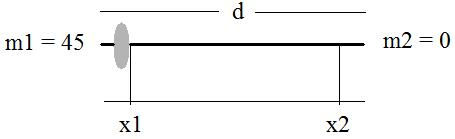If you find any of this useful, please consider donating via PayPal to help keep this site going.# Barbell Tipping

12/12/11

In bodybuilding/strength training forums I regularly read this question "How much weight can I add to one side of a racked barbell before it tips over?" There are a lot of simplifying assumptions one can make to tackle this problem, and here is a general idea. Statics experts (of which I am not one) - please write in!

Let a racked barbell that weighs B lbs have its supports at x1 and x2. Say there is m1 lbs at x1 and m2 lbs at x2. The center of mass of the unweighted barbell itself is at d/2, where d is the length of the barbell.

Note that for a bar to not be tipping, it is not rotating about a support. Therefore the sum of the torque equals zero. Mathematically, for an arbitrary point X, this is

sum(torques) = m1*g*(X-x1)+m2*g*(X-x2)

Note that if sum(torques) = 0, this implies that X = Xcm (center of mass), and

Xcm = (m1x1+m2x2)/(m1+m2)

I've ignored the weight, B lbs, of the bar here. Adding that back in, we get

Xcm = (B*d/2 + m1x1+m2x2)/(B+m1+m2)

So when will the barbell tip over? Without loss of generality, assume we are just adding weight to m1. The bar will then tip when Xcm < x1. That is, when the center of mass is outside of the support. The situation is:Note something interesting here. Because each weight plate has a thickness, the new x1 point that we are loading with mass is actually shifting to a number smaller than x1 as we add additional weight to the bar. So we're not just adding more weight, but adding more weight further from the support.

If I substitute in specific numbers, these tend to match reality pretty well. For around 3 to 3+ plates it tends to tip for the setups I've used if there are no plates on the other side (ie. m2 = 0). Here's a setup with some hypothetical numbers:

• B = 45 lbs
• bar's center of mass = d/2 = 42 inches
• starting m1 = 45 lbs
• m2 = 0 lbs
• starting x1 = 24 inches
• x2 = 60 inches
• assume x1 shifts 3 inches to the left of the starting x1 with each plate added

Note that for 1 and 2 plates added to m1, Xcm is still greater than 24 inches (the original x1). However, when a little over 3 plates are added, Xcm < 24, and therefore tipping occurs.

In practice, however, I'd recommend to not ever have the difference between m1 and m2 be large at all. Actually, there's really no need to use a barbell in the first place.

Standard exercise disclaimer: The material contained in this article is provided for informational purposes only. Before undertaking any form of exercise, you should consult with your physician. You should be aware if you engage in exercise you are doing so entirely at your own risk, including any present and/or future physical or psychological pain or injury that you may incur. The author of this content cannot assume any responsibility or liability for any injuries or losses that you may incur as a result of acting upon any information provided by this content, or any links to other exercise information found herein.

If you enjoyed any of my content, please consider supporting it in a variety of ways: